# Random Walk (1d) – C PROGRAM

In the last post I wrote about how to simulate a coin toss/flip using random numbers generated within the range:$[0,1]$.

We can use that code to simulate a popular stochastic process, called the random walk.
NOTE: This will also serve as a test for our random number generator.

Let’s consider an elementary example of a 1-dimensional random walk on a number line. The walker starts at 0 and can take a step forward (positive increment) or a step backward (-ve increment), both with equal probabilities.

We know that for an unbiased coin the probability of getting a Heads or Tails is equal. I’ve already written about it in the last post. So we will just use that code for the flip of the coin, which will decide whether our random walker moves forward or backward.

So, let’s write a program that simulates a random walk and plot the distance travelled versus the number of steps taken. This plot will let us verify/confirm if our program truly depicts a random walk or not.

### CODE:

/*********************************
RANDOM WALK 1-d
Plot the path of a 1-d random walker and print out the final displacement
*********************************/
#include<stdio.h>
#include<math.h>
/**Function that generates a random number.
Parameters:
r0: initial (first) seed
a: scale factor , so that a*r0 give the first random number
m: gives the max. value of random numbers that can be generated (m-1)
c: additional displacement factor
**/
int rand(int r0, int a, int m, int c){
int r1=(a*r0+c)%m;
return r1;
}
/**Function that generates random numbers given a seed, and stores them in an array that is passed as an argument.
Parameters:
r0: initial (first) seed
a: scale factor , so that a*r0 give the first random number
m: gives the max. value of random numbers that can be generated (m-1)
c: additional displacement factor
n: no. of random numbers to be generated
x[n]: array that will store the random numbers
**/
void randomNos(int r0, int a, int m, int c, int n, int x[n]){
int r1=rand(r0,a,m,c);;
int i;
for(i=0;i<n;i++){
x[i]=r1;
r1=rand(r1,a,m,c);
}
}
/**Function that results the result of a coin toss:
Parameters:
r: a random number between 0 and 1
Returns 1 for Heads and 0 for tails
**/
int coinTossSingle(double r){
if(r>0.5){
return 1;
} else if(r<0.5){
return 0;
}
}
/**Function that generates n coin tosses results, given a seed and other starting conditions, and stores them in an array that is passed as an argument.
Parameters:
r0: initial (first) seed
a: scale factor , so that a*r0+c give the first random number
m: gives the max. value of random numbers that can be generated (m-1)
c: additional displacement factor
n: no. of coin tosses to be generated
x[n]: array that will store the random numbers
**/
void coinToss(int r0, int a, int m, int c, int n, int results[n]){
int randNos[n];
randomNos(r0, a, m, c, n, randNos);
//Renormalize to 0 to 1
int i;
double randNosNew[n];
for(i=0;i<n;i++){
randNosNew[i]=(double)randNos[i]/(m-1);
}
for(i=0;i<n;i++){
results[i]=coinTossSingle(randNosNew[i]);
}

}
main(){
int a, m, c, r0, n;
printf("Enter the value of a:\n");
scanf("%d",&a);
printf("Enter the value of m:\n");
scanf("%d",&m);
printf("Enter the value of c:\n");
scanf("%d",&c);
printf("Enter the value of r0(initial):\n");
scanf("%d",&r0);
printf("Enter the no. of steps require:\n");
scanf("%d",&n);
int tossResults[n];
coinToss(r0, a, m, c, n, tossResults);
int i;
//Step-size
double h=1;
//Origin (Start of random walk)
double x0=0,origin=x0;
double x1;
//Array to store the position of the random walker at the ith step
double x[n];
for(i=0;i<n;i++){
if(tossResults[i]==1){
x1=x0+h;
} else{
//Tails=>Move left
x1=x0-h;
}
//Store the position at the ith step in array x[i]
x[i]=x1;
x0=x1;
}
//Plot the random Walk (Trajectory)
FILE *fp=NULL;
fp=fopen("randomWalk1.txt","w");
for(i=0;i<n;i++){
fprintf(fp,"%d\t%lf\n",i+1,x[i]);
}
double dist=x1-origin;
printf("\nThe distance travelled is:\n%lf",dist);
}


### OUTPUT:

The above plots seems a good example for a random walker from a naïve perspective, so we can now move further and work on more problems on 1-d random walk.

Now, let’s use the above program to verify some commonly known properties of a random walker, which are,

1. The expectation value of distance travelled by a 1-d random walker is 0.
Consider a random walker that starts from origin, and we let it take$N$ steps, and note down it’s distance travelled ($d$) from the origin after$N$ steps. Repeat this process$M$ times, and take the average of the$d$ values that you get. For infinite$M$ you would get$\langle d \rangle = 0$.

2. The expectation value of the square of the distance travelled by a 1-d random walker after$N$ steps, is$N$$\langle d^2 \rangle=N$
or$\sqrt{\langle d^2 \rangle }=\sqrt{N}$
The above quantity is called the root mean squared distance, and it is roughly the distance that we can expect our random walker to have walked after N steps.

So, let’s modify the above program and add a few more lines to perform the calculations for a$\langle d \rangle$ and$\langle d^2 \rangle$.

What I’ll do is, I’ll run the above random walk simulation, for different number of steps$n$ from 0 to 1,000 in steps of 100. For each value of$n$, the random walk simulation is run$M$ times. Therefore, there is the variable M in the code initialized as 100000, to run the random walk simulation$100,000$ times.

To make each of the M runs of the simulation different from each other, we will need a different and completely randomly chosen seed for each run. So at the end of each of M runs I generate a new random number form the last seed from the previous iteration. Then I have the arrays d and d2 that will store the value of$d$ and$d^2$ for each of the M runs. Finally I’ve just calculated the averages of the values stored in d and d2, and stored them in a .txt file along with the value of n. So that we can plot$d$ and$d^2$ vs.$N$.

### CODE:

/*********************************
RANDOM WALK 1-d
Plot <d(N)> and <d^2(N)> vs N
*********************************/
#include<stdio.h>
#include<math.h>
/**Function that generates a random number.
Parameters:
r0: initial (first) seed
a: scale factor , so that a*r0 give the first random number
m: gives the max. value of random numbers that can be generated (m-1)
c: additional displacement factor
**/
int rand(int r0, int a, int m, int c){
int r1=(a*r0+c)%m;
return r1;
}
/**Function that generates random numbers given a seed, and stores them in an array that is passed as an argument.
Parameters:
r0: initial (first) seed
a: scale factor , so that a*r0 give the first random number
m: gives the max. value of random numbers that can be generated (m-1)
c: additional displacement factor
n: no. of random numbers to be generated
x[n]: array that will store the random numbers
**/
void randomNos(int r0, int a, int m, int c, int n, int x[n]){
int r1=rand(r0,a,m,c);;
int i;
for(i=0;i<n;i++){
x[i]=r1;
r1=rand(r1,a,m,c);
}
}
/**Function that results the result of a coin toss:
Parameters:
r: a random number between 0 and 1
Returns 1 for Heads and 0 for tails
**/
int coinTossSingle(double r){
if(r>0.5){
return 1;
} else{
return 0;
}
}
/**Function that generates n coin tosses results, given a seed and other starting conditions, and stores them in an array that is passed as an argument.
Parameters:
r0: initial (first) seed
a: scale factor , so that a*r0+c give the first random number
m: gives the max. value of random numbers that can be generated (m-1)
c: additional displacement factor
n: no. of coin tosses to be generated
x[n]: array that will store the random numbers
**/
void coinToss(int r0, int a, int m, int c, int n, int results[n]){
int randNos[n];
randomNos(r0, a, m, c, n, randNos);
//Renormalize to 0 to 1
int i;
double randNosNew[n];
for(i=0;i<n;i++){
randNosNew[i]=(double)randNos[i]/(m-1);
}
for(i=0;i<n;i++){
results[i]=coinTossSingle(randNosNew[i]);
}

}
main(){
int a=1093, m=86436, c=18257, n, r0=43, M=100000, stepCount=0, N=1000;
//int m=121500, a=1021,c=25673, n, r0=51,M=100000, stepCount=0, N=1000;
//int m=259200, a=421, c=54773, n, r0=12, M=100000, stepCount=0, N=1000;
//int m=121500, a=2041, c=25673, n, r0=25, M=100000, stepCount=0, N=1000;

/*printf("Enter the value of a:\n");
scanf("%d",&a);
printf("Enter the value of m:\n");
scanf("%d",&m);
printf("Enter the value of c:\n");
scanf("%d",&c);
printf("Enter the value of r0(initial):\n");
scanf("%d",&r);*/

FILE *fp="NULL";
fp=fopen("randomWalk4.txt","w");
double d[M];
double d2[M];
//Run the random-walk simulation for n steps
for(n=0;n<=N;n=n+100){
printf("%d\n",stepCount); //To keep trak of where we are in the execution
stepCount++; //To keep trak of where we are in the execution
int j;
//Run the same simulation M times
for(j=0;j<M;j++){
int tossResults[n];
//use the coin toss/flip result to define forward or backward movement
coinToss(r0, a, m, c, n, tossResults);
int i;
double h=1;
double x0=0,origin=0;
double x1;
int count;
count=0;
count=0;
for(i=0;i<n;i++){
if(tossResults[i]==1){
//x1=x0+h;
count++;
} else{
//	x1=x0-h;
count++;
}
//x0=x1;
}
//find the distance from origin
//d[j]=x1-origin;
d[j]=count-count;
//square of the distance
d2[j]=pow(d[j],2);
//generate a new seed at each of the M runs
r0=rand(r0,a,m,c);

}
//find out the averages of the d and d^2 after M runs
double sum1=0,sum2=0;
for(j=0;j<M;j++){
sum1=sum1+d[j];
sum2=sum2+d2[j];
}
double dav=sum1/M;	// <d>
double dav2=sum2/M; // <d^2>
//store the value of n, <d> and <d^2> in .txt file for each n
fprintf(fp,"%d\t%lf\t%lf\n",n,dav,dav2);
}
}


### OUTPUT:

#### —>For a=1093, m=86436, c=18257,

when we plot the data from the text file generated after the execution, we get the following plots.
For N=1000, M=100,000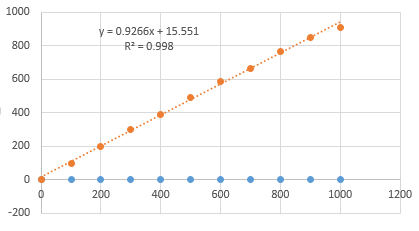Expectation value of d and d^2 vs N1-d Random Walk

But, for N=5000, M=100,000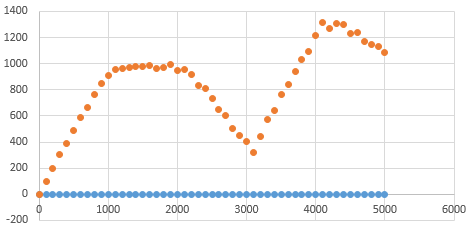Expectation value of d and d^2 vs N1-d Random Walk

Here we something interesting happening.
The value of$\langle d^2 \rangle$ for N(the number of steps taken)>1000 we don’t get the expected results. This implies that our random number generator is not ideal. There are some correlations.

Remember, we our using the following algorithm to generate random numbers,$r_{i+1}=(a \times r_i+c)\mathrm{mod } m$
called the Linear Congruential Generator
This algorithm generates a maximum of$(m-1)$ random numbers with the maximum value of$m-1$ (Try to see why is it so).
Here,$r_i$ is the seed.
The values of$a$,$m$ and$c$ are carefully chosen values.

Here we have an option to change the values of a,m and c.
Mathematicians have tested many values for these and here, I am writing a few of those taken from Numerical Recipes in C.
1. m=86436, a=1093, c=18257
2. m=121500, a=1021,c=25673
3. m=259200, a=421, c=54773
4. m=121500, a=2041, c=25673

Let’s run the above code again for different values of a,m and c and see the results.

#### —> For m=121500, a=1021, c=25673, we get

For N=2000, M=100,000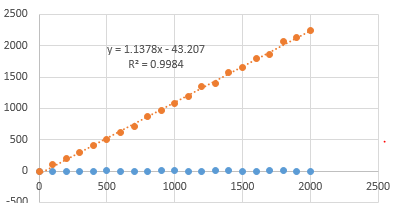Expectation value of d and d^2 vs N1-d Random Walk
For N=10000, M=10,000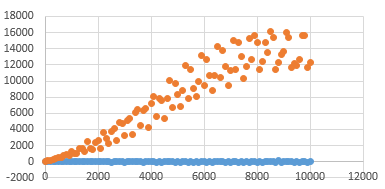Expectation value of d and d^2 vs N1-d Random Walk

#### —>For m=259200, a=421, c=54773, we get

N=5000, M=100,000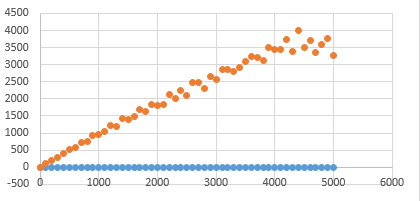Expectation value of d and d^2 vs N1-d Random Walk

N=10,000, M=10,000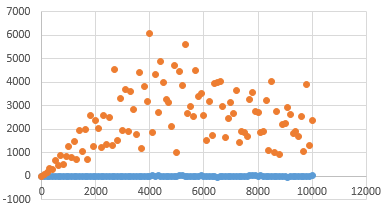Expectation value of d and d^2 vs N1-d Random Walk

#### —>For m=121500, a=2041, c=25673, we get

For$0\leq n\leq N=2200$, M(Trials)=100000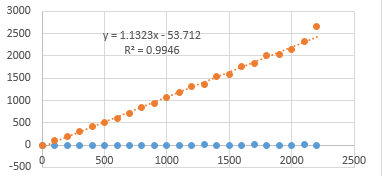Expectation value of d and d^2 vs N1-d Random Walk
For$0\leq n\leq N=5000$, M(Trials)=10000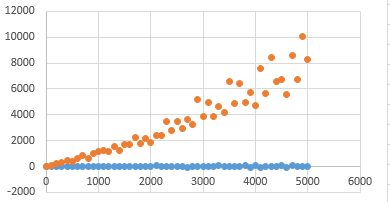Expectation value of d and d^2 vs N1-d Random Walk
We see that for different values we get the expected value of$\langle d^2 \rangle$ for larger N.

But still, we are not getting the expected behaviour for N larger than, 2000.

So, maybe we need a better random number generator. Or maybe we can try to use an even more random seed by using the system clock.

Let’s try that.

### References:

http://www.mit.edu/~kardar/teaching/projects/chemotaxis(AndreaSchmidt)/random.htm

http://mathworld.wolfram.com/RandomWalk1-Dimensional.html

https://en.wikipedia.org/wiki/Random_walk[wpedon id="7041" align="center"]